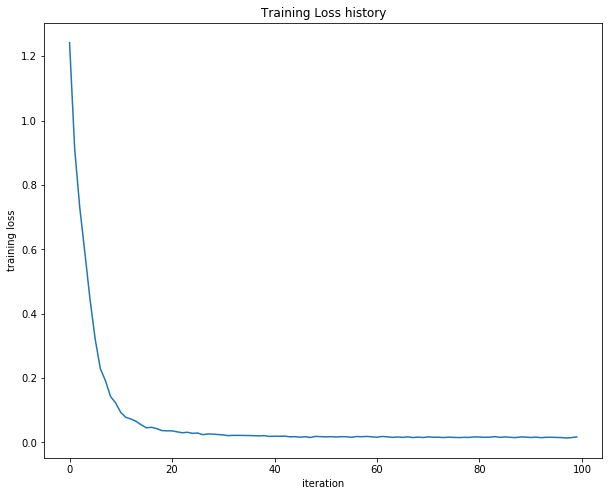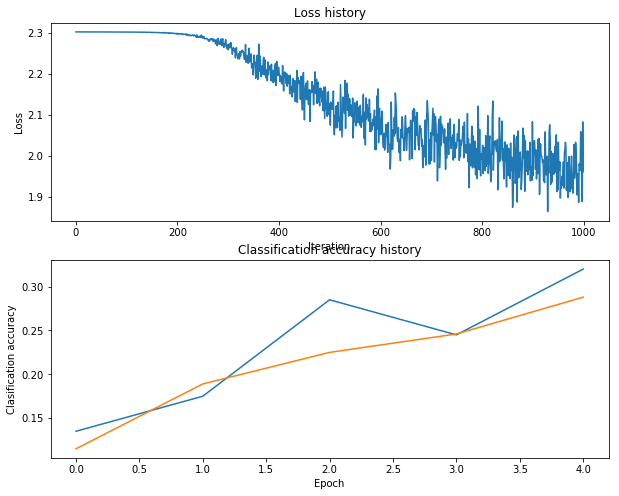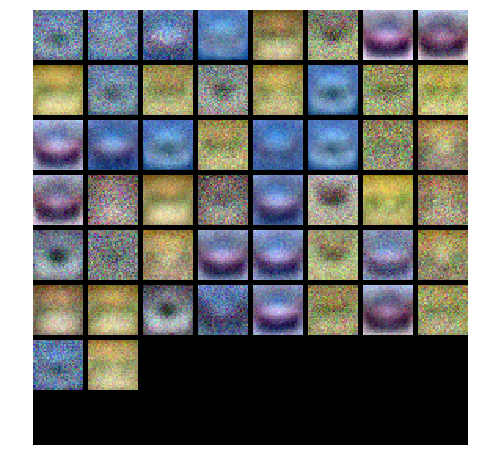# Neural Netork之python实现

Posted by jjx on March 12, 2017

    W1, b1 = self.params['W1'], self.params['b1']
W2, b2 = self.params['W2'], self.params['b2']
N, D = X.shape

# Compute the forward pass
scores = None

hidden_layer = np.maximum(0, np.dot(X, W1) + b1) #ReLU
scores = np.dot(hidden_layer, W2) + b2
# print scores

# If the targets are not given then jump out, we're done
if y is None:
return scores

# Compute the loss
loss = None

# visual prob
exp_scores = np.exp(scores)
probs = exp_scores / np.sum(exp_scores, axis=1, keepdims=True) # [N x K]

#calculate loss
corect_logprobs = -np.log(probs[range(N),y])
data_loss = np.sum(corect_logprobs)/N
reg_loss = 0.5*reg*np.sum(W1*W1) + 0.5*reg*np.sum(W2*W2)
loss = data_loss + reg_loss

dscores = probs
dscores[range(N),y] -= 1
dscores /= N

# graident backforwar
dW2 = np.dot(hidden_layer.T, dscores)
db2 = np.sum(dscores, axis=0, keepdims=False)

dhidden = np.dot(dscores, W2.T)

dhidden[hidden_layer <= 0] = 0
dW1 = np.dot(X.T, dhidden)
db1 = np.sum(dhidden, axis=0, keepdims=False)

dW2 += reg * W2
dW1 += reg * W1



def rel_error(x, y):
""" returns relative error """
return np.max(np.abs(x - y) / (np.maximum(1e-8, np.abs(x) + np.abs(y))))

print 'Difference between your scores and correct scores:'
print np.sum(np.abs(scores - correct_scores))

loss, _ = net.loss(X, y, reg=0.1)
correct_loss = 1.30378789133

# should be very small, we get < 1e-12
print 'Difference between your loss and correct loss:'
print np.sum(np.abs(loss - correct_loss))

loss, grads = net.loss(X, y, reg=0.1)

# these should all be less than 1e-8 or so

f = lambda j: net.loss(X, y, reg=0.1)
#print type(f),type(net.loss(X, y, reg=0.1))


def train(self, X, y, X_val, y_val,
learning_rate=1e-3, learning_rate_decay=0.95,
reg=1e-5, num_iters=100,
batch_size=200, verbose=False):
num_train = X.shape
iterations_per_epoch = max(num_train / batch_size, 1)

# Use SGD to optimize the parameters in self.model
loss_history = []
train_acc_history = []
val_acc_history = []

for it in xrange(num_iters):
X_batch = None
y_batch = None

idx = np.random.choice(num_train, batch_size, replace=True)
X_batch = X[idx]
y_batch = y[idx]

# Compute loss and gradients using the current minibatch
loss, grads = self.loss(X_batch, y=y_batch, reg=reg)
loss_history.append(loss)

if verbose and it % 100 == 0:
print 'iteration %d / %d: loss %f' % (it, num_iters, loss)

# Every epoch, check train and val accuracy and decay learning rate.
if it % iterations_per_epoch == 0:
# Check accuracy
train_acc = (self.predict(X_batch) == y_batch).mean()
val_acc = (self.predict(X_val) == y_val).mean()
train_acc_history.append(train_acc)
val_acc_history.append(val_acc)

# Decay learning rate
learning_rate *= learning_rate_decay

return {
'loss_history': loss_history,
'train_acc_history': train_acc_history,
'val_acc_history': val_acc_history,
}

def predict(self, X):
y_pred = None

hidden_layer = np.maximum(0, np.dot(X, self.params['W1']) + self.params['b1'])
scores = np.dot(hidden_layer, self.params['W2']) + self.params['b2']
y_pred = np.argmax(scores, axis=1)
return y_pred


net = init_toy_model()
stats = net.train(X, y, X, y,
learning_rate=1e-1, reg=1e-5,
num_iters=100, verbose=False)

print 'Final training loss: ', stats['loss_history'][-1]

# plot the loss history
plt.plot(stats['loss_history'])
plt.xlabel('iteration')
plt.ylabel('training loss')
plt.title('Training Loss history')
plt.show()Train data shape:  (49000, 3072)
Train labels shape:  (49000,)
Validation data shape:  (1000, 3072)
Validation labels shape:  (1000,)
Test data shape:  (1000, 3072)
Test labels shape:  (1000,)


input_size = 32 * 32 * 3
hidden_size = 50
num_classes = 10
net = TwoLayerNet(input_size, hidden_size, num_classes)

# Train the network
stats = net.train(X_train, y_train, X_val, y_val,
num_iters=1000, batch_size=200,
learning_rate=1e-4, learning_rate_decay=0.95,
reg=0.5, verbose=True)

# Predict on the validation set
val_acc = (net.predict(X_val) == y_val).mean()
print 'Validation accuracy: ', val_acc


#### Debug the training

One strategy for getting insight into what’s wrong is to plot the loss function and the accuracies on the training and validation sets during optimization.
Another strategy is to visualize the weights that were learned in the first layer of the network. In most neural networks trained on visual data, the first layer weights typically show some visible structure when visualized.

# Plot the loss function and train / validation accuracies
plt.subplot(2, 1, 1)
plt.plot(stats['loss_history'])
plt.title('Loss history')
plt.xlabel('Iteration')
plt.ylabel('Loss')

plt.subplot(2, 1, 2)
plt.plot(stats['train_acc_history'], label='train')
plt.plot(stats['val_acc_history'], label='val')
plt.title('Classification accuracy history')
plt.xlabel('Epoch')
plt.ylabel('Clasification accuracy')
plt.show()def show_net_weights(net):
W1 = net.params['W1']
W1 = W1.reshape(32, 32, 3, -1).transpose(3, 0, 1, 2)
plt.gca().axis('off')
plt.show()

show_net_weights(net)What’s wrong?. Looking at the visualizations above, we see that the loss is decreasing more or less linearly, which seems to suggest that the learning rate may be too low. Moreover, there is no gap between the training and validation accuracy, suggesting that the model we used has low capacity, and that we should increase its size. On the other hand, with a very large model we would expect to see more overfitting, which would manifest itself as a very large gap between the training and validation accuracy.

Tuning. Tuning the hyperparameters and developing intuition for how they affect the final performance is a large part of using Neural Networks, so we want you to get a lot of practice. Below, you should experiment with different values of the various hyperparameters, including hidden layer size, learning rate, numer of training epochs, and regularization strength. You might also consider tuning the learning rate decay, but you should be able to get good performance using the default value.

hidden_size = [75, 100, 125]

results = {}
best_val_acc = 0
best_net = None

learning_rates = np.array([0.7, 0.8, 0.9, 1, 1.1])*1e-3
regularization_strengths = [0.75, 1, 1.25]

print 'running',
for hs in hidden_size:
for lr in learning_rates:
for reg in regularization_strengths:
print '.',
net = TwoLayerNet(input_size, hs, num_classes)
# Train the network
stats = net.train(X_train, y_train, X_val, y_val,
num_iters=1500, batch_size=200,
learning_rate=lr, learning_rate_decay=0.95,
reg= reg, verbose=False)
val_acc = (net.predict(X_val) == y_val).mean()
if val_acc > best_val_acc:
best_val_acc = val_acc
best_net = net
results[(hs,lr,reg)] = val_acc
print
print "finshed"
# Print out results.
for hs,lr, reg in sorted(results):
val_acc = results[(hs, lr, reg)]
print 'hs %d lr %e reg %e val accuracy: %f' % (hs, lr, reg,  val_acc)

print 'best validation accuracy achieved during cross-validation: %f' % best_val_acc


best validation accuracy achieved during cross-validation: 0.502000.

test_acc = (best_net.predict(X_test) == y_test).mean()
print 'Test accuracy: ', test_acc